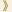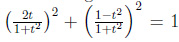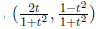HomeShaw Laureates2017Mathematical SciencesEssay

Since ancient times, a central theme in mathematics has been the study of polynomials and their solutions. Algebraic geometry is the study of the properties of sets of solutions to polynomial equations in several variables. A simple example of such an equation is x2+y2+z2 = 1, the solution set of which is the surface of a sphere of radius 1.

As this example demonstrates, solution sets of polynomial equations, which are known as varieties, are geometric objects. Examining the interplay between the algebra and the geometry has turned out to be remarkably fruitful, and algebraic geometry is a major branch of mathematics, the study of which has profound consequences not just for algebra and geometry but also for several other areas ranging from number theory to mathematical physics.

For each type of mathematical structure, there is normally a notion of when two examples are essentially the same. For varieties, the notion is called birational equivalence: two varieties are said to be birationally equivalent if, after removing lower-dimensional subvarieties if necessary, there is a rational map from one to the other with a rational inverse. (A rational map is a ratio of two polynomials.) Rational varieties are those that are birationally equivalent to ordinary n-dimensional space, for some n. To give an example, for every real number t one can check that, from which it follows that the map that takes a real number t to the pointsends an infinite line to the unit circle. One can check further that sending a point (x, y) in the unit circle to the real number (1 - y) /x inverts this map. There is a small problem here in that no value of t maps to the point (0, -1), but this point on its own is a zero-dimensional subset, which we are allowed to ignore. Thus, a circle is birationally equivalent to ordinary 1-dimensional space, which means that it is a rational variety.

Some of the most exciting advances in algebraic geometry over the past few decades have been in better understanding the birational classification of higher-dimensional varieties. For example, Shigefumi Mori won the Fields Medal for his “Minimal Model Program”, which attempts to find in each birational equivalence class a unique simplest variety. There have also been very important breakthroughs in characterizing rational varieties: that is, in finding ways of telling whether a variety is rational.

Along with his work on birational classification, Kollár’s most recent work stands out in a direction that will greatly affect algebraic geometry in the decades to come, as an important complement of the Minimal Model Program: the definition and study of moduli of higher-dimensional varieties, which can be thought of as sophisticated geometrical structures whose points represent equivalence classes of these varieties. The importance of this area can be seen in the immense wealth of papers on the moduli problem in dimension 1, which today occupies topologists, combinatorialists and, perhaps most of all, physicists. Already for surfaces the treatment of moduli is an extremely subtle and difficult problem. Kollár’s ideas have almost defined the field of higher-dimensional moduli.

Among Voisin’s major achievements is the solution of the Kodaira problem, which starts with the observation that every deformation of a complex projective manifold is a Kähler manifold (which roughly speaking means a geometric set that locally has a structure compatible with the complex numbers) and asks whether the converse is true. She found counterexamples: that is, Kähler manifolds that do not just fail to be deformations of projective manifolds but are not even topologically equivalent to projective manifolds. Another of Voisin’s pioneering accomplishments is the establishment a new technique for showing that a variety is not rational, a breakthrough that has led to results that would previously have been unthinkable. A third remarkable result is a counterexample to an extension of the Hodge conjecture, one of the hardest problems in mathematics (it is one of the Clay Mathematical Institute’s seven Millennium Problems), which rules out several approaches to the conjecture.

Algebraic geometry is a central area of mathematics that has seen many remarkable developments in its history, and these developments continue to the present day. In their different ways, János Kollár and Claire Voisin have made profound contributions to algebraic geometry that will deeply influence the future of the subject.

26 September 2017   Hong Kong